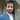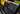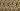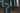Navigate back to the homepage

# ReasonML - Advent of Code - Day 1Amit Solanki
December 1st, 2019 · 4 min readAdvent of Code, is an advent calendar to solve small programming puzzles, every day for 25 days. once you solve one puzzle the next one opens up.

Signup for Advent Of Code 2019 if you haven’t already, and please try and attempt it before reading this post, especially if you are a beginner. We are going to attempt it in ReasonML

### Intro to ReasonML

Reasonml is a typed language which compiles down to Javascript. To get more intro on it I would recommend you to visit the official getting started guide.

I am only going to introduce the language features which we are going to use and should be aware of.

• To define a variable we use the `let` keyword followed by the variable name.
`.css-1chxjt6{position:absolute;right:22px;top:24px;padding:8px 12px 7px;border-radius:5px;color:#6f7177;-webkit-transition:background 0.3s ease;transition:background 0.3s ease;}.css-1chxjt6:hover{background:rgba(255,255,255,0.07);}.css-1chxjt6[data-a11y="true"]:focus::after{content:"";position:absolute;left:-2%;top:-2%;width:104%;height:104%;border:2px solid var(--theme-ui-colors-accent,#6166DC);border-radius:5px;background:rgba(255,255,255,0.01);}@media (max-width:45.9375em){.css-1chxjt6{display:none;}}1let counter = 0;`
• Types are inferred. This means that we don’t have to write types for every variable we use. In the above example the variable `counter` is of type `int`.

• If a variable is of type `int` (integer) we cannot do any operations which needs a `float` (Floating number). To do any operation using that variable we will have to type cast (convert) it into another type, which is float in this example.

`1let floatingCounter = float_of_int(counter);`
• Array is defined like so
`1let inputArray = [|1, 2, 3, 4|]`
• Notice we use `|` after `[` and before `]`. This is to differentiate between array (similar to Javascript arrays) and List which is just ``. We will focus on array for now.
• We define functions like so.
`1let myFunction = (param) => {2  // function body ...3  param4}`
• Last statement of a function is the return value.
• If else statement is to be used for ternaries. By this I mean that the last statement in their block is the return value of the block, for example.
`1let initialValue = 10;2let counter =3  if (initialValue <= 10) {4    5;5  } else {6    10;7  };`
• We don’t need to import any files here. Files are automatically scoped as modules in ReasonML.
• Reasonml can be converted to Ocaml and Javascript. We use BuckleScript to convert ReasonML to Javascript, which provides us some handy utility functions from JS-land in Reason, like `Map`, `Reduce`, `Filter` etc. We will be using `Map` and `Reduce` from `Belt` which is a stdlib shipped with BuckleScript.
• To log using `console.log` we can use `Js.log`

### Setup

We want a bare minimum setup, in which we can create a file for each day and run it to get the solution. We will run the generated JS file in Node.

`1npm install -g bs-platform # to install buckle-script2bsb -init advent-of-code -theme basic-reason # initialise or project3cd advent-of-code4yarn add nodemon # nodemon helps us reload node server on file changes`

We’ll need a few scripts to get output on every change, add the following in you `package.json`.

`1"scripts": {2    "nodemon": "nodemon ./src/index.bs.js",3    "build": "bsb -make-world",4    "start": "bsb -make-world -w",5    "clean": "bsb -clean-world"6  }`

The files generated by BuckleScript has `.bs` before them and are readable like normal JS (they don’t uglify it), we can edit them but I wouldn’t recommend at this stage.

There are two scripts of interest here. `start` and `nodemon`. `start` will generate `.bs.js` files for us whenever we make change in `.re` (reasonml) files. `nodemon` will restart the `node` process whenever `index.bs.js` file changes or any of it’s imported files change.

Rename the `Demo.re` file in src to `Day1.re` and create a function `main` in it, like so.

`1let main = () => {2  Js.log("Hello World")3}`

Lets create our point of entry file, that is the `index.re` which will generate `index.bs.js`. Create the file in `src`. We need to call our `main` function in `Day1.re` from `index.re`, in reasonml there is no need to import from other files, they are scoped with their file names so to call `main` function in `index.re` we can directly call it as `Day1.main()`. So our `index.re` file should look like.

`1Day1.main()`

Now let’s look at the first problem.

### First Problem

We are given a list of masses, and need to calculate the fuel needed for each one of them and report the total amount of fuel needed. Fuel needed for each item of mass `m` if given by `mass / 3 - 2`.

To solve the given problem, we will loop through each item in the list(array) calculate the fuel values, then add them to get the final result.

Let’s define some test values in an array.

`1let test_values = [| 14, 9, 12 |]`

Now we need a function to calculate the fuel for a given mass. This function will take an `int` divide it `3`, `floor` the result and subtract `2` from it.

`1let fuel_calculator = (mass) => {2  let mass_as_float = float_of_int(mass); // we need mass in float since floor accepts only floating values3  let mass_divided_by_three = mass_as_float /. 3.0; // notice the division sign and 3 as 3.04  let floored_value = floor(mass_divided_by_three);5  let floored_value_as_int = int_of_float(floored_value)6  let fuel_value = floored_value_as_int - 2;7  fuel_value // the return value8}`

Here we first convert the integer input to float, using `float_of_int` function. Then we divide by `3.0`, for denoting a value as float we have to add `.0` at the end. Then we floor the value using `floor` function, this is similar to `Math.floor` in JS. The floored value is a `float` and we want `int` to proceed, so we convert it to `int` using `in_of_floor`. Then we just subtract the value with `2`.

let’s call the function to test if it works properly.

`1Js.log(fuel_calculator(14)) // should log '2'`

let’s refactor the function a bit.

`1let fuel_calculator = mass => {2  int_of_float(floor(float_of_int(mass) /. 3.0)) - 2;3};`

We can use the `|>`(pipe) operator to pipe the value from one function to another. let’s see how we can use it in the above function.

`1let fuel_calculator = mass => {2  ((mass |> float_of_int) /. 3.0 |> floor |> int_of_float) - 2;3};`

You can read the above function as take mass and convert it to float, divide by 3, floor it, convert it back to int and subtract 2 from the result.

Now that we have a way to calculate the fuel, let’s look into looping over the array. Just as in javascript we use `Array.map` to map over an array to get another array, we can use `Belt.Array` to loop over an array. (I am assuming that you are aware of JS Map).

We can use it like below.

`1let mapping_function = (array_value) => array_value2let result = Belt.Array.map(input_array, mapping_function)`

Since it would be tedious to write `Belt.Array` every time we use it, let’s alias it.

`1module A = Belt.Array2let mapping_function = (array_value) => array_value3let result = A.map(input_array, mapping_function)`

It would also be better if we can just pipe the array into Map like we did before. `|>` is used to pipe the last argument, and `->` is used to pipe the first argument.

`1module A = Belt.Array2let mapping_function = array_value => array_value;3let result = input_array->A.map(mapping_function);`

Great, now we know how to map and we have the mapping function as well as the input, let’s combine all of them together

`1module A = Belt.Array;23let fuel_calculator = mass => {4  ((mass |> float_of_int) /. 3.0 |> floor |> int_of_float) - 2;5};67let initialValue = [|14, 9, 12|];89let main = () => {10  let result = initialValue->A.map(fuel_calculator);11  result |> Js.log;12}`

Console should log `[2, 1, 2]`. Now we have an array of fuel values for each mass, we need to add all of them to get final result.

To do so we will use `Array.reduce`. and an add function. The add function takes 2 values `i` and `j` adds them and returns the value. (I am asumming you know Array.reduce from JS)

`1module A = Belt.Array;23let fuel_calculator = mass => {4  ((mass |> float_of_int) /. 3.0 |> floor |> int_of_float) - 2;5};67let initialValue = [|14, 9, 12|];89let add = (i, j) => i + j;1011let main = () => {12  let result = initialValue->A.map(fuel_calculator)->A.reduce(add);13  result |> Js.log;14}`

Console should log `5`. Now you can pass the input you get from Advent Of code to get your solution.

### Second Problem

Now we have to calculate the mass of the fuel we are adding, and the fuel required to add that fuel, and so on and so forth. This looks like a classic recursive call problem. let’s see how we can approach it in ReasonML.

we first need to calculate the fuel required to carry the said mass, then we have to calculate the fuel required to carry that Fuel, and recursively call until the mass is 0.

`1let rec getTotalFuel = mass => {2  if (mass <= 0) {3    0;4  } else {5    let fuelReq = getFuel(mass);6    if (fuelReq <= 0) {7      mass;8    } else {9      getTotalFuel(fuelReq) + mass;10    };11  };12};`

Notice we have added `rec` after let, to say that this function is a recursive function. Let’s refactor it a bit, we can do away with just one if-else here. Since we know that any value less than 9 will mean fuel required is 0.

`1let rec get_total_fuel = mass => {2  if (mass < 9) {3    mass;4  } else {5    let fuelReq = getFuel(mass);6    get_total_fuel(fuelReq) + mass;7  };8};`

The above function should give us our result, now we need to run it for each mass-fuel value.

`1module A = Belt.Array;23let fuel_calculator = mass => {4  ((mass |> float_of_int) /. 3.0 |> floor |> int_of_float) - 2;5};67let initialValue = [|14, 9, 12|];89let add = (i, j) => i + j;1011let rec get_total_fuel = mass => {12  if (mass < 9) {13    mass;14  } else {15    let fuelReq = getFuel(mass);16    get_total_fuel(fuelReq) + mass;17  };18};1920let main = () => {21  let result = initialValue->A.map(fuel_calculator)->A.map(get_total_fuel)->A.reduce(add);22  result |> Js.log;23}`

Et voilà, we have our solution. We can refactor the above functions, like tail call optimisation etc. We will explore more tomorrow. Mesage me or comment here if you have any question.

### More articles from Amit Solanki### Optional Chaining for Javascript - ES proposal

Understanding the Optional chaining operator, an ES features which is moved to stage 3.

November 30th, 2019 · 1 min read### Nullish Coalescing for JavaScript

Understanding the Null coalescing operator, an ES features which is moved to stage 3.

November 29th, 2019 · 1 min read